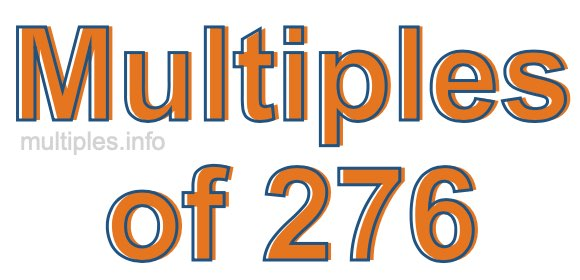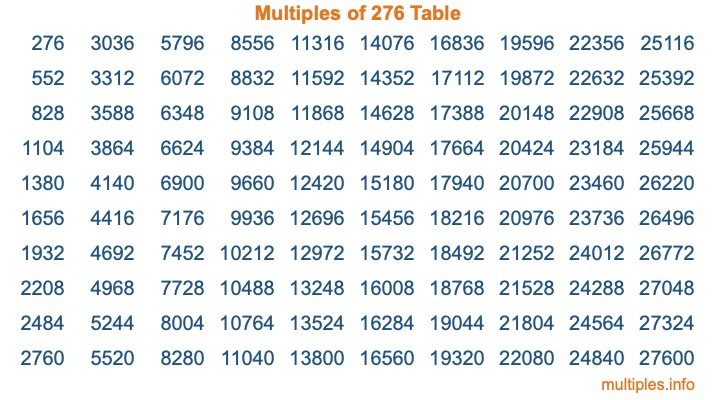Multiples of 276Welcome to the Multiples of 276 page. Here we will first teach you everything you will ever need to know about the multiples of 276, and then give you a study guide summary of everything we taught you to make sure you remember it all. Use this page to look up facts and learn information about the multiples of 276. This page will make you a multiples of two hundred seventy-six expert!

Definition of Multiples of 276
Multiples of 276 are all the numbers that when divided by 276 equal an integer. Each of the multiples of 276 are called a multiple. A multiple of 276 is created by multiplying 276 by an integer.

Therefore, to create a list of multiples of 276, you start with 1 multiplied by 276, then 2 multiplied by 276, then 3 multiplied by 276, and so on for as long as you want. Thus, the list of the first five multiples of 276 is 276, 552, 828, 1104, and 1380. To see a larger list of multiples of 276, see the printable image of Multiples of 276 further down on this page. We also have a category where you can choose any nth multiple of 276.

Multiples of 276 Checker
The Multiples of 276 Checker below checks to see if any number of your choice is a multiple of 276. In other words, it checks to see if there is any number (integer) that when multiplied by 276 will equal your number. To do that, we divide your number by 276. If the the quotient is an integer, then your number is a multiple of 276.

Is  a multiple of 276?

Least Common Multiple of 276 and ...
A Least Common Multiple (LCM) is the lowest multiple that two or more numbers have in common. This is also called the smallest common multiple or lowest common multiple and is useful to know when you are adding our subtracting fractions. Enter one or more numbers below (276 is already entered) to find the LCM.

Check out our LCM Calculator if you need more details about the Least Common Multiple or if you need the LCM for different numbers for adding and subtraction fractions.

nth Multiple of 276
As we stated above, 276 is the first multiple of 276, 552 is the second multiple of 276, 828 is the third multiple of 276, and so on. Enter a number below to find the nth multiple of 276.

th multiple of 276

Multiples of 276 vs Factors of 276
276 is a multiple of 276 and a factor of 276, but that is where the similarities end. All postive multiples of 276 are 276 or greater than 276. All positive factors of 276 are 276 or less than 276.

Below is the beginning list of multiples of 276 and the factors of 276 so you can compare:

Multiples of 276: 276, 552, 828, 1104, 1380, etc.

Factors of 276: 1, 2, 3, 4, 6, 12, 23, 46, 69, 92, 138, 276

As you can see, the multiples of 276 are all the numbers that you can divide by 276 to get a whole number. The factors of 276, on the other hand, are all the whole numbers that you can multiply by another whole number to get 276.

It's also interesting to note that if a number (x) is a factor of 276, then 276 will also be a multiple of that number (x).

Multiples of 276 vs Divisors of 276
The divisors of 276 are all the integers that 276 can be divided by evenly. Below is a list of the divisors of 276.

Divisors of 276: 1, 2, 3, 4, 6, 12, 23, 46, 69, 92, 138, 276

The interesting thing to note here is that if you take any multiple of 276 and divide it by a divisor of 276, you will see that the quotient is an integer.

Multiples of 276 Table
Below is an image of the first 100 multiples of 276 in a table. The table is in chronological order, column by column. The first column has the first ten multiples of 276, the second column has the next ten multiples of 276, and so on.The Multiples of 276 Table is also referred to as the 276 Times Table or Times Table of 276. You are welcome to print out our table for your studies.

Negative Multiples of 276
Although not often discussed or needed in math, it is worth mentioning that you can make a list of negative multiples of 276 by multiplying 276 by -1, then by -2, then by -3, and so on, to get the following list of negative multiples of 276:

-276, -552, -828, -1104, -1380, etc.

Multiples of 276 Summary
Below is a summary of important Multiples of 276 facts that we have discussed on this page. To retain the knowledge on this page, we recommend that you read through the summary and explain to yourself or a study partner why they hold true.

There are an infinite number of multiples of 276.

A multiple of 276 divided by 276 will equal a whole number.

276 divided by a factor of 276 equals a divisor of 276.

The nth multiple of 276 is n times 276.

The largest factor of 276 is equal to the first positive multiple of 276.

276 is a multiple of every factor of 276.

276 is a multiple of 276.

A multiple of 276 divided by a divisor of 276 equals an integer.

276 divided by a divisor of 276 equals a factor of 276.

Any integer times 276 will equal a multiple of 276.

Multiples of a Number
Here you can get the multiples of another number, all with the same attention to detail as we did for multiples of 276 on this page.

Multiples of
Multiples of 277
Did you find our page about multiples of two hundred seventy-six educational? Do you want more knowledge? Check out the multiples of the next number on our list!